#The Model Student: A Rube-ish Square (Part 2)

Overload Journal #90 - April 2009 + Programming Topics + Design of applications and programs   Author: Richard Harris
A rube-ish square embodies some simple group theory. Richard Harris explores its properties.

In the previous article in this series, presumably to counter a lifetime of the humiliation of being the only person in my socially maladjusted peer group unable to solve Rubik's Cube, I introduced the Rube-ish Square; a two dimensional version of that fiendish puzzle that even I might be able to solve. The Rube-ish Square is manipulated by rotating its rows and columns, with the element pushed out being returned on the opposite side, as illustrated in figure 1.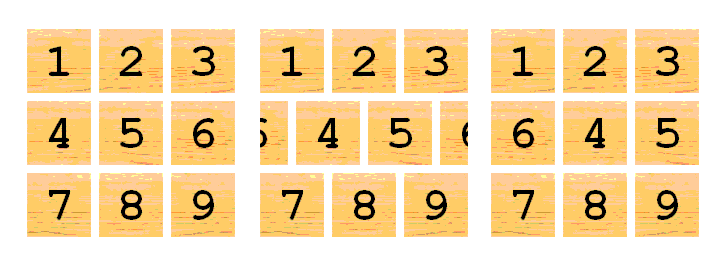Figure

As you will no doubt recall, in an unforgivably maths heavy discourse, we exploited group theory to describe the properties of this simplified version of the cube. Remember that a group is defined as a set of elements together with an operator (usually denoted by ) which satisfies the following rules: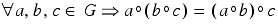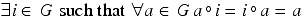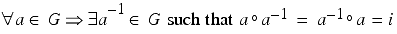Since they are not common programming notation, I feel that I should probably remind you that the upside down A means for all, the backwards E means there exists and the rounded E means within.

So translating this formal definition into English again, these rules mean that for a group G: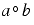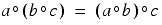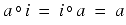Specifically we showed that the Rube-ish Square is fully described by the group representing the permutations of a vector of nine elements that involve swapping an even number of pairs of elements; namely the alternating group of degree nine.

Hence we were able to show that the Rube-ish Square has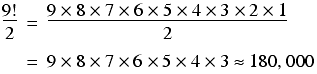possible states.

This time we shall address a question that still remains unanswered for Rubik's Cube; what is the largest number of moves ever required to return the square to its initial state? And for this, as promised, we shall abandon the harsh mistress of mathematics and return to the warm safe embrace of C++.

## Make with the C++ already

The first things we're going to need are some classes to represent the board and our interactions with it.

Listing 1 illustrates the move_type structure that we shall use to indicate how we wish to manipulate the square. The decision to use a structure rather than a class reflects this type's intended use to simply bind together the three pieces of data describing a move; the direction, the id of the row or column and the count of how many squares to the left or right, n. The constructors are straightforward, as shown in Listing 2.

 ``` enum direction { horizontal, vertical, }; struct move_type { direction dir; size_t id; long n; move_type(); move_type(direction dir, size_t id, long n); }; ``` Listing 1

 ``` move_type::move_type() { } move_type::move_type( direction dir, size_t id, long n) : dir(dir), id(id), n(n) { } ``` Listing 2

The default constructor is required since we will eventually want to store objects of this type in a standard sequence container and it leaves the member data uninitialised since they have no meaningful default values.

Listing 3 illustrates the board class that we will use to represent the state of the Rube-ish square and manipulate it.

 ``` class board { public: typedef size_t value_type; typedef std::vector board_type; typedef board_type::const_iterator const_iterator; board(); explicit board(size_t n); size_t size() const; void move(move_type m); void undo(move_type m); const board_type & data() const; const_iterator begin() const; const_iterator end() const; value_type at(size_t row, size_t col) const; private: void move_row(size_t row, long n); void move_col(size_t col, long n); value_type & at(size_t row, size_t col); board_type board_; board_type buffer_; }; ``` Listing 3

The first thing to note is that by enabling construction with different lengths of side n this class represents a more general puzzle than the one we are currently investigating. The move and undo member functions provide the means to manipulate the state of the square, and the remaining public member functions the means to observe it.

The private member functions provide the state manipulation mechanism that will be used by both move and undo.

Finally, the member data board_ and buffer_ represent the board itself and a temporary area to assist in the manipulation of its rows and columns.

Listing 4 illustrates the implementation of the constructors.

 ``` board::board() { } board::board(size_t n) : board_(n*n, 0), buffer_(n, 0) { static const size_t max_n = 1UL<<( std::numeric_limits::digits/2); if(n>=max_n) throw std::invalid_argument(""); size_t i = 1; board_type::iterator first = board_.begin(); board_type::iterator last = board_.end(); while(first!=last) *first++ = i++; } ``` Listing 4

The default constructor need do nothing since both the member data have default constructors that do what we require; namely create a container of length 0.

The initialising constructor is of slightly more interest. This initialises the board with n2 elements that will hold the row-wise elements of the board and initialises the working buffer with n elements that will hold the elements of a single row or column whilst they are being manipulated. It then proceeds to fill the board with integers counting up from 1 to create the initial, solved, state.

The size member function returns the length of side of the square and exploits the fact that the number of elements in the working buffer is equal to the number of rows and columns, as illustrated below:

```      size_t
board::size() const
{
return buffer_.size();
}

```

The state access member functions are fairly straightforward, generally simply forwarding on to the underlying data type, as illustrated in Listing 5. The p1most complex of these, the at member function, simply maps the row and column onto a row-wise element of the board_ member, throwing an exception if an attempt is made to access an element out of the board's limits.

 ``` const board::board_type & board::data() const { return board_; } board::const_iterator board::begin() const { return board_.begin(); } board::const_iterator board::end() const { return board_.end(); } board::value_type board::at(size_t row, size_t col) const { if(row>=size() || col>=size()) throw std::out_of_range(""); assert(row*size()+col

The move and undo member functions simply forward on the requests to manipulate the state of the board to the private member functions move_row and move_col as shown in Listing 6.

 ``` void board::move(move_type m) { if(m.dir==horizontal) move_row(m.id, m.n); else move_col(m.id, m.n); } void board::undo(move_type m) { if(m.dir==horizontal) move_row(m.id, -m.n); else move_col(m.id, -m.n); } ``` Listing 6

As you can see, undo is simply the opposite operation to a move. Listing 7 illustrates how the private member functions perform the required state manipulation.

 ``` void board::move_row(size_t row, long n) { if(row>=size()) throw std::out_of_range(""); if(n<0) n = size() - (-n)%size(); for(size_t i=0;i!=size();++i) buffer_[i] = at(row, i); for(size_t j=0;j!=size();++j) at(row, (j+n)%size()) = buffer_[j]; } void board::move_col(size_t col, long n) { if(col>=size()) throw std::out_of_range(""); if(n<0) n = size() - (-n)%size(); for(size_t i=0;i!=size();++i) buffer_[i] = at(i, col); for(size_t j=0;j!=size();++j) at((j+n)%size(), col) = buffer_[j]; } board::value_type & board::at(size_t row, size_t col) { assert(row

So we can see that the move_row and move_col member functions simply copy the row or column to be manipulated into the working buffer and then copy the elements back into the board starting from the required offset, using modular arithmetic to ensure that we correctly wrap them around the left and right or top and bottom.

## We've got a Rube-ish square and we're not afraid to use it

So, now we have the implementation of the board we are ready to use it to investigate the question of what is the largest number of moves required to return the square to its initial state from any other state.

To answer this, we shall represent the 180,000 or so states of the square as nodes, each of which is connected to 12 others by lines representing the 12 distinct rotations of rows and columns with which we transform the square from one state to another.

The technical name for this kind of structure is a graph, although the term network is also occasionally used. Figure 2 provides an illustration of a small graph of just 9 nodes.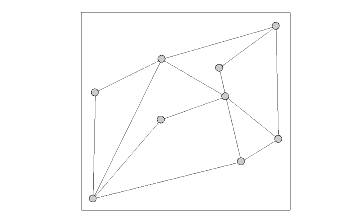Figure 2

Graphs played a big role in the early days of artificial intelligence [Russell95] , where they were used to represent the states of an artificial world, often a game such as chess, and the actions that transform it between states. A number of algorithms were developed to efficiently search these graphs for a series of transformations that would lead from an initial to a desired state.

Unfortunately, the real world rarely provides us with such well-defined states and transformations and so these algorithms never led to the goal of machines that demonstrate intelligent behaviour. Within the field of computer gaming, however, we often do have rigidly defined rules and goals and consequently these types of algorithms still form the basis for many game playing machines. A particularly striking example is IBM's chess playing machine, Deep Blue, which defeated world champion Gary Kasparov in 1997 by searching through some 200 million states per second [Hsu02] .

If we disallow returning to a node already visited, the paths through a graph may be represented by a simpler structure; namely a tree. Numbering the nodes in our example graph, the first three levels of the tree of all paths starting at the uppermost node are illustrated in Figure 3.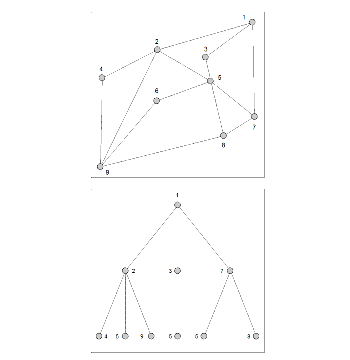Figure 3

Strictly speaking, we could also represent paths that allowed nodes to be revisited with a tree, although if we wished to examine all possible paths it would need an infinite number of levels.

Structuring the problem in this form is highly suggestive of a way to search the paths through the graph. Starting at the first node we can recursively descend the leftmost branch until we reach the bottom, then reverse up a level and descend the next leftmost, and so on until we have traversed every non-returning path starting at the first node. Figure 4 illustrates the full 9 steps of the search.Figure 4

This approach is known as depth-first search, and is one of the simpler graph traversal algorithms. Its principal drawback is that, when searching for a path to a node with a particular property, it often follows a large number of fruitless paths before discovering a relatively short one that has the desired result. Specifically, if the rightmost branch leads to a node with the desired property after one step, depth-first search is going to waste a lot of time on the leftmost branches.

As a result, it is generally not the algorithm of choice for many problems. That said, the alternatives require maintaining a great deal more state, trading computational expense for memory usage.

The first thing we should note is that , so we can generate a unique identifier for any given state of the 3 by 3 square that fits inside a 32 bit integer. The scheme we shall use is to represent the state as a 9 digit base 9 number:where nij is the number in row i and column j of the square in the given state. Note that we must subtract 1 from each element since they range from 1 to 9, rather than from 0 to 8.

Listing 8 illustrates the addition of such an id member function to the board class.

 ``` class board { public: ... unsigned long id() const; ... }; unsigned long board::id() const { if(size()>3) throw std::out_of_range(""); unsigned long i = 0; const_iterator first = begin(); const_iterator last = end(); while(first!=last) { i *= size()*size(); i += *first++ - 1; } return i; } ``` Listing 8

This scheme is fairly wasteful of bits, since for the 3 by 3 square can only exhibit even permutations of the integers from 1 to 9; approximately 218.47 states. We could certainly do better by identifying each permutation with no gaps, which would allow us to use a sequential rather than an associative container to record the set of already visited states. Unfortunately, this would be rather more complicated to implement.

Furthermore, as you may have guessed from the first line of the function, it won't work for board sizes greater than 3. This is because the result of the formula won't fit into a 32 bit integer if the board size is 4 or greater.

In fact, even the total number of possible states won't fit into a 32 bit integer for a 4 by 4 board, so the more efficient scheme wouldn't do us any good either. For a 5 by 5 board, we wouldn't even have enough space if we had 64 bits to work with.

Figure 5 illustrates just how quickly the number of states and the upper bound of our encoding scheme grow with the size of the board, n..

n

States

Encoding

2

25

28

3

217

229

4

244

264

5

283

2116

6

2138

2186

Figure 5

Clearly we're going to run out of bits pretty quickly no matter how large our integers are. Giving up the encoding scheme and using vectors of integers to represent the board state doesn't help much either since instead of running out of bits, we'll run out of memory.

Given the enormous difficulty we will clearly have representing the full set of states of large boards, I'm reasonably happy to accept the weaknesses of this encoding scheme. In any event, the board we are studying is just 3 by 3, so it's something of a moot point.

So, all that said, exactly how inefficient is a depth first search going to be?

Unfortunately, it's going to be pretty damn well inefficient since we may very well visit all 180,000 states during traversal of the leftmost sub-tree of the root node, only to replace them with shorter paths later in the search.

Thankfully, we can dramatically improve our algorithmic performance by setting an upper bound on the depth of the tree.

To do this, we need only describe a naïve scheme for solving the puzzle; namely find the worst case for returning each number to its initial position in turn, whilst leaving previously returned numbers in place. I shall simply assert what these worst cases are and how many moves they take, so you may wish to take a moment to confirm that I've got them all right.

• For 1, the worst case is when it is in neither the first row nor the first column, requiring 2 moves.
• For 2, whilst leaving the 1 in the correct position, the worst case is if it is in the first row and third column, requiring 3 moves to ensure that 1 remains in the correct position.
• For 3, whilst leaving the 1 and the 2 in the correct positions, the worst case is when it is in neither the first row nor the third column, requiring 2 moves.
• For 4, whilst leaving the previous elements in their correct positions, the worst case is if it is in the third row and the first column, requiring 4 moves.
• For 5, whilst leaving the previous elements in their correct positions, the worst case is if it is in the third row and the second column, requiring 4 moves.
• For 6, whilst leaving the previous elements in their correct positions, the worst case is if it is in the third row and the third column, requiring 4 moves.
• For 7, whilst leaving the previous elements in their correct positions, the worst case is if it is in the second or third column, requiring 1 move.
• At this point, 8 and 9 must be in their correct positions since if they weren't we'd have an odd permutation of the square and, as we so tediously demonstrated last time, that just isn't possible.

So, that gives us an upper bound of just 20 moves, significantly reducing the worst case number of branches we must traverse.

Adding a typedef for a std::map from the board state id to the number of moves required to get there from the initial state to the board, we are ready to begin searching for the state that requires the most moves to return the square to its initial state.

## The final piece of the puzzle

Listing 9 illustrates the definition of the record of moves and the declaration of the max_moves static member function that we'll use to find the state requiring the most moves to solve.

 ``` class board { public: ... static size_t max_moves(size_t n, size_t hint = 0); ... private: typedef std::map move_counts_type; ... }; ``` Listing 9

Note that the hint argument will be used to inform the algorithm of any upper bound on the depth of the search tree that we have been able to deduce. For our analysis this will of course be 20 moves. We'll use the default value of 0 as an indication that we haven't done our homework and are unaware of any such upper bound.

The max_moves function simply initialises a board and a move count and forwards on to another function, examining its result for the worst case, as illustrated in Listing 10.

 ``` size_t board::max_moves(size_t n, size_t hint) { move_counts_type move_counts; board b(n); all_moves(b, move_counts, 0, hint); move_counts_type::const_iterator first = move_counts.begin(); move_counts_type::const_iterator last = move_counts.end(); size_t result = 0; while(first!=last) { if(first->second>result) result = first->second; ++first; } return result; } ``` Listing 10

So, the real work is being done by the as yet undefined all_moves function, given in Listing 11.

 ``` class board { ... private: static void all_moves(board &b, move_counts_type &move_counts, size_t depth, size_t hint); ... }; void board::all_moves(board &b, move_counts_type &move_counts, size_t depth, size_t hint) { size_t id = b.id(); move_counts_type::value_type current_count(id, depth); std::pair current = move_counts.insert(current_count); if((current.second || depthsecond) && (hint==0 || depth<=hint)) { if(!current.second) current.first->second = depth; next_moves(b, move_counts, depth, hint); } } ``` Listing 11

This simply checks whether the current state of the board has been visited before and if not, or if so but after a greater number of moves, adds it to the record of states and the number of moves required to reach them. It then recursively takes a further step by calling the next_moves member function, which in turn relies upon a next_move function, both of which are illustrated in Listing 12.

 ``` class board { ... private: static void next_move(board &b, move_type m, move_counts_type &move_counts, size_t depth, size_t hint); static void next_moves(board &b, move_counts_type &move_counts, size_t depth, size_t hint); ... }; void board::next_move(board &b, move_type m, move_counts_type &move_counts, size_t depth, size_t hint) { b.move(m); all_moves(b, move_counts, depth+1, hint); b.undo(m); } void board::next_moves(board &b, move_counts_type &move_counts, size_t depth, size_t hint) { for(size_t i=0;i!=b.size();++i) { for(size_t n=1;n!=b.size();++n) { move_type h(horizontal, i, n); move_type v(vertical, i, n); next_move(b, h, move_counts, depth, hint); next_move(b, v, move_counts, depth, hint); } } } ``` Listing 12

So next_moves simply iterates over the rows and columns of the board and calls next_move to continue the search with each move that can be applied to them. Note that the number of squares through which we rotate the rows and columns by starts at 1 rather than 0, since the latter would trivially have no effect upon the square. We don't have to worry about the inner loop running into trouble if the board has a size of 0, since in that case the outer loop will terminate immediately.

Now we are finally ready to calculate the worst case number of moves required to solve our Rube-ish Square, and upon doing so we discover that it is, rather anti-climactically, 8.

Before I leave you again, I thought it might be rather fun to give you a couple of examples of 8-move states of the square in Figure 6, so that you can have a crack at solving them.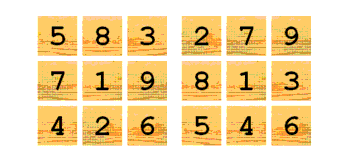Figure 6

As a final thought, I'd like you to imagine that the elements of the square are also labelled on the underside and that, in addition to rotating its rows and columns, we allow the whole square to be turned over, swapping the 1st and 3rd, the 4th and 6th and the 7th and 9th pieces. This is an odd permutation, so trivially doubles the number of possible states. The question is, what effect does it have on the worst case number of moves?

Until next time, dear reader, happy puzzling.